Next: Phase Transformations in Van Up: Multi-Phase Systems Previous: Phase Diagrams

# Vapor Pressure

The Clausius-Clapeyron equation can be used to derive an approximate expression for the pressure of the vapor in equilibrium with the liquid (or solid) at some temperature. This pressure is called the vapor pressure of the liquid (or solid) at this temperature. According to Equation (9.74),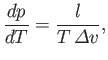(9.75)

where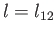is the latent heat per mole, and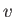the molar volume. Let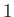refer to the liquid (or solid) phase, and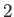to the vapor. It follows that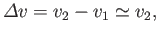(9.76)

because the vapor is much less dense than the liquid, so that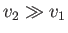. Let us also assume that the vapor can be adequately treated as an ideal gas, so that its equation of state is written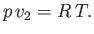(9.77)

Thus,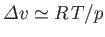, and Equation (9.75) becomes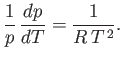(9.78)

Assuming that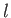is approximately temperature independent, we can integrate the previous equation to give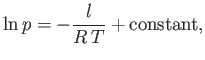(9.79)

which implies that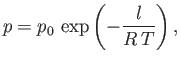(9.80)

where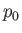is some constant. This result shows that the vapor pressure,, is a very rapidly increasing function of the temperature,.Next: Phase Transformations in Van Up: Multi-Phase Systems Previous: Phase Diagrams
Richard Fitzpatrick 2016-01-25